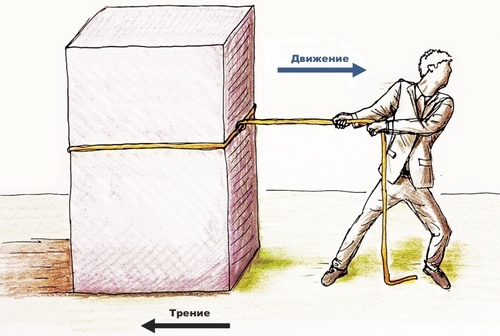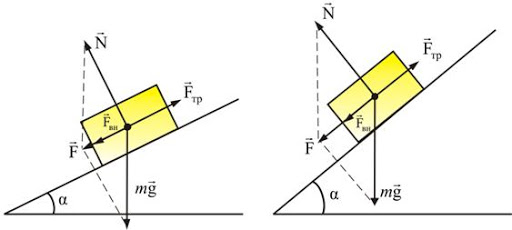# Friction Force in Physics: Definition, FormulasContent:

• Friction Definition

• Types of Frictional Force

• Types of Friction

• How to Calculate the Friction Force?

• Friction Force Formula

• Friction Force, Video

The friction force arises from the contact of the surfaces of two physical bodies. The theory of friction worried the minds of mankind, since the earliest times. Ancient engineers: builders of the Egyptian pyramids, Stonehenge in England or mysterious stone idols on Easter Island, all of them (as well as their modern colleagues) were dealing with the problem of friction and how to minimize it. It is difficult to move heavy loads due to the friction force. Our distant ancestors invented such a useful invention as the wheel and many other important discoveries to solve this problem. In our article, we will look at the friction force in the physical aspect.

## Friction Definition

What is frictional force in physics? The classic definition is as follows: friction force is a force that appears when two bodies come into contact during motion and impede this motion itself. The greater the friction force between the bodies, the more difficult it is to move them relative to each other. What causes the force of friction? In physical meaning, friction appears as a result of the interaction between atoms and molecules of bodies that are in contact with each other.

Newton’s third law acts on the bodies during the friction: the friction force acting on the first body (body A) is equal to the friction force acting on the second body (body B). Only these forces have the opposite direction in absolute value.Look at this picture. The friction force acting on the refrigerator is equal to the friction force acting on the floor. Yet these forces are directed in opposite directions.

## Types of Frictional Force

There are such types of friction forces as:

• Static. The force of static friction occurs when two bodies come into contact, which, however, do not move relative to each other. The force of static friction has a zero value.
• Sliding. The force of sliding friction is the most classic illustration of the action of friction, which occurs when the bodies slip relative to each other. Its value is affected by body weight (the larger it is, the greater the friction force), the nature of the surface (of course, when sliding on ice the friction force will be several times less than when sliding on the ground).
• Rolling. The force of rolling friction appears when one body rolls on the surface of another, for example, when riding a bicycle or a car. The friction force is much less during rolling than during sliding.
• Twists. The spinning friction force is manifested when one body rotates on the surface of another.## Types of Friction

• Dry – appears in contact with hard surfaces.
• Viscous, also similar friction is called liquid friction, appears when a solid touches a liquid or gas. For example, viscous (liquid) friction acts on a ship sailing on the water, as well as on the surface of the water. The force of viscous friction is usually much less than the force of dry friction.
• Mixed, appears when there is a layer of lubricant between the surfaces that come into contact.

An interesting fact: during the siege of Constantinople in 1453, the Turks pulled their ships overland to bypass the special chain that blocked the way for Turkish ships to the Golden Horn. To reduce the friction force when moving large heavy warships, they made flooring made of wooden rails, which was abundantly greased with lard. The Turks successfully realized their plan, bringing the defenders of Constantinople into genuine confusion.Sultan Mehmed II oversees the transport of his ships.

As you can see, knowledge of the laws of physics and mechanics more than once or twice found its practical embodiment in real life.

Let us return from the history again to physics. Friction may be external and internal. External friction is characteristic of the interaction of exclusively solid bodies. Internal friction is characterized by viscosity and occurs during the interaction of liquids or gases. Such an interaction can occur inside a conditionally single body. For example, there are different currents, with colder or warmer water in the waters of the oceans, when these currents interact with each other, internal friction arises.

## How to Calculate the Friction Force?

It is necessary to know the coefficient of friction k (or µ), which depends on the nature of the surface to calculate the friction force. The coefficient of friction is a constant value. The coefficient of friction can be found in a special table.In addition to the coefficient of friction, it is necessary to know the force of the reaction of the surface N, which, in essence, is equal to the force of gravity depending on the mass of the body (m) and the acceleration of gravity. Its formula will be as follows:

N = m * g

Where m is the mass of the body and g is the acceleration of gravity, this is a constant value of 9.8 m/s2.

## Friction Force Formula

The friction force is calculated by multiplying the reaction of the surface N and the coefficient of friction k. The formula of the friction force will have the following form:

Ffr = k * N.

In some formulas, the coefficient of friction k is denoted by the symbol µ.

The above calculations are valid in the simplest case when the body lies on a strictly horizontal surface.If the friction motion occurs along an inclined plane, then the calculations of the friction force are somewhat more complicated. The body is affected by the force of gravity and the reaction of the resistance of the surface, but not in one direction.So the formula of the friction force for a body that moves along an inclined surface will have the following form:

Ffr = k * m * g * cosα.

Where k is the coefficient of friction, m is the mass of the body, g is the gravitational constant (remember that it is 9.8 m/s2), cosα is the ratio of the cathetus adjacent to the corner to the hypotenuse of the triangle (cosine).

The connection between physics and geometry is clearly manifested in calculating the friction force on inclined surfaces.

• Beer, Ferdinand P.; Johnston, E. Russel, Jr. (1996). Vector Mechanics for Engineers (Sixth ed.). McGraw-Hill. p. 397. ISBN 978-0-07-297688-5.
• Jump up to: a b Meriam, J. L.; Kraige, L. G. (2002). Engineering Mechanics (fifth ed.). John Wiley & Sons. p. 328. ISBN 978-0-471-60293-4.
• Ruina, Andy; Pratap, Rudra (2002). Introduction to Statics and Dynamics (PDF). Oxford University Press. p. 713.
• Hibbeler, R. C. (2007). Engineering Mechanics (Eleventh ed.). Pearson, Prentice Hall. p. 393. ISBN 978-0-13-127146-3.
• Soutas-Little, Robert W.; Inman, Balint (2008). Engineering Mechanics. Thomson. p. 329. ISBN 978-0-495-29610-2.

## Friction Force, Video### Author: Pavlo Chaika, Editor-in-Chief of the journal Poznavayka

When writing this article, I tried to make it as interesting and useful as possible. I would be grateful for any feedback and constructive criticism in the form of comments to the article. You can also write your wish/question/suggestion to my mail pavelchaika1983@gmail.com or to Facebook.